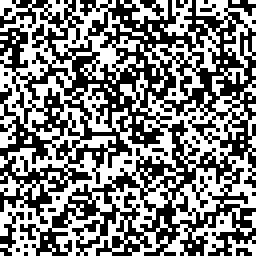Instantly share code, notes, and snippets.

# kaityo256/test.cpp

Created May 10, 2021 10:18
C++ to Python Sample
This file contains bidirectional Unicode text that may be interpreted or compiled differently than what appears below. To review, open the file in an editor that reveals hidden Unicode characters. Learn more about bidirectional Unicode characters
 #include #include #include #include #include const int L = 64; const int N = L * L; std::vector spins(L *L); void init() { std::mt19937 mt; std::uniform_int_distribution<> ud(0, 1); for (int i = 0; i < N; i++) { int r = ud(mt); spins[i] = r * 2 - 1; } } void save_data(const std::string filename) { std::ofstream ofs(filename, std::ios::binary); ofs.write((char *)spins.data(), spins.size() * sizeof(int)); } int main() { init(); save_data("test.dat"); }

### kaityo256 commented May 10, 2021

`test.py`

```from PIL import Image, ImageDraw
import numpy as np

with open("test.dat", "rb") as f:
spins = np.frombuffer(data, dtype='<i')

L = 64
g = 4
im = Image.new("RGB", (L*g, L*g), "white")
draw = ImageDraw.Draw(im)

color = "black"
for x in range(L):
for y in range(L):
i = x + y*L
if spins[i] == 1:
color = "black"
else:
color = "white"
draw.rectangle((x*g, y*g, x*g+g, y*g+g), fill=color)

im.save("test.png")```

### kaityo256 commented May 10, 2021

```g++ test.cpp
./a.out
python test.py```

test.pngができます。